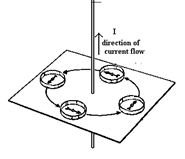# Physics - Online Test

Q1. Work done by a uniform electric field E in moving a charge q a distance d from a to b is
Explaination / Solution:

Force and the work done
Q2. The basic property of a fluid that makes it different from solids
Explaination / Solution:

fluids can flow due to unbalanced forces between the atoms of fluids.

Q3. Kinetic theory explains the behavior
Explaination / Solution:

The theory for ideal gases makes the following assumptions 1. Gases consist of particles in constant, random motion. They continue in a straight line until they collide with something—usually each other or the walls of their container. 2. Particles are point masses with no volume. The particles are so small compared to the space between them, that we do not consider their size in ideal gases. 3. No molecular forces are at work. This means that there is no attraction or repulsion between the particles. 4. Gas pressure is due to the molecules colliding with the walls of the container. All of these collisions are perfectly elastic, meaning that there is no change in energy of either the particles or the wall upon collision. No energy is lost or gained from collisions. 5. The time it takes to collide is negligible compared with the time between collisions. 6. The kinetic energy of a gas is a measure of its Kelvin temperature. Individual gas molecules have different speeds, but the temperature and kinetic energy of the gas refer to the average of these speeds. 7. The average kinetic energy of a gas particle is directly proportional to the temperature. An increase in temperature increases the speed in which the gas molecules move. 8. All gases at a given temperature have the same average kinetic energy. 9. Lighter gas molecules move faster than heavier molecules.

Q4. What is the percentage of the mass of an atom concentrated in the nucleus?
Explaination / Solution:

A proton and a neutron have roughly 2000 times the mass of an electron. The numbers of an electron in a stable atom (not isotope) is equal to the electrons. So for most atoms, the nucleus will hold roughly 4000 times the mass of its electron cloud. Thus,
Q5. Light rays from a point object
Explaination / Solution:
No Explaination.

Q6. A wire is perpendicular to the plane of the paper. A ring of compass needles surrounds the wire in the plane of the paper with center of ring being the center of the wire .Initially there is no current in the wire. What happens after a steady dc current is established in the wire?
Explaination / Solution:

The current carrying wire has a magnetic field around it and the lines of force are in the form of concentric circles with their centers on the wire.Q7. Heat is a form of
Explaination / Solution:

Heat is the amount of energy flowing from one body of matter to another spontaneously due to their temperature difference.

Q8. Physical quantities are
Explaination / Solution:

A physical quantity is a physical property of a phenomenon, body, or substance, that can be quantified by measurement.A physical quantity can be expressed as the combination of a magnitude expressed by a number – usually a real number – and a unit. All these given above can be expressed as explained so these are physical quantity.

Q9. According to Mendel alleles have the following character
Explaination / Solution:
No Explaination.

Q10. The linear momentum and position vector of the planet is perpendicular to each other at
Explaination / Solution:
No Explaination.